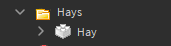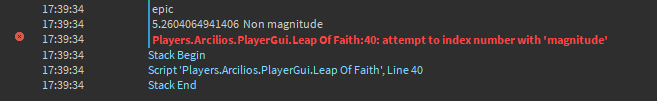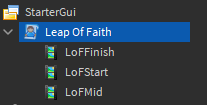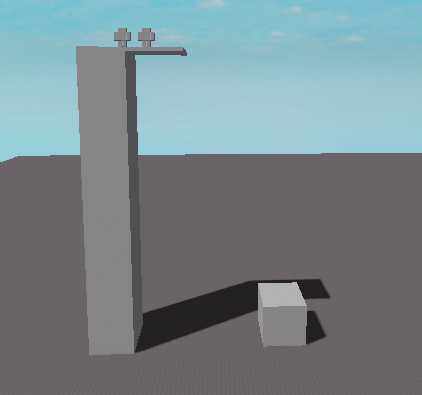# Magnitude not working?

Hello,

I’ve been working with magnitude recently to make a Leap Of Faith affect from Assassin Creed, so when I started writing the script I had to check the Z and the Y axis between the player and the hay stacks to make sure the player is above the hay stacks on a high spot, but this is when I ran into this problem magnitude is not working with 1 Axis nor all axis and I’m confused to why this is happening, any help is appreciated.

Hay stacks folder:Error:Script:``````local UIS = game:GetService("UserInputService")
local player = game.Players.LocalPlayer
local character = player.Character or player.CharacterAdded:Wait()

repeat wait() until character:FindFirstChild("Humanoid")

local Count = 0

-- Checking if the player is running, crouching, etc
local function CheckBehavior()
local folder = character.Behavior
for i, v in ipairs(folder:GetChildren()) do
if v:IsA("BoolValue") then
if v.Value == false then
Count += 1
end
end
end
end

UIS.InputBegan:Connect(function(inpt, UPE)
if UPE then return end

if inpt.KeyCode == Enum.KeyCode.F then
CheckBehavior()
if Count == 4 then
Count = 0
print("epic")
-- Looping through the hays and comparing positions
for i, v in ipairs(game.Workspace.Hays:GetChildren()) do
-- Getting the magnitude between the player and the hay stack
local UpMag = (player.Character.HumanoidRootPart.Position.Y - v.Position.Y)
local DisMag = (player.Character.HumanoidRootPart.Position.Z - v.Position.Z)
print(DisMag, " Non magnitude")
print(DisMag.magnitude, " with magnitude") -- Error here
print(UpMag.magnitude) -- Error here

if DisMag.magnitude <= 30 and UpMag.magnitude >= 20 then
print("Check")
local Root = player.Character.HumanoidRootPart
local Info = TweenInfo.new(3,Enum.EasingStyle.Quint,Enum.EasingDirection.Out)
character.Humanoid.Jump = true
-- Pulling the player to the hay stack
game:GetService("TweenService"):Create(Root, Info, {Position = v.Position}):Play()
print("Started")
end
end
end
end
end)
``````You can only use `magnitude` on Vector3 Values. Since you’re getting only a part of the Vector3 values when indexing `UpMag` and `DisMag`, it’s just a number, hence `attempt to index number with magnitude`.

``````local StartPoint = Vector3.new(0,0,0)
local EndPoint = Vector3.new(100,100,100)

local Magnitude = (EndPoint - StartPoint).magnitude
``````

`player.Character.HumanoidRootPart.Position.Y - v.Position.Y`
The `Y` values are plain numbers and what this returns is also a plain number.
`UpMag.magnitude`
There’s no magnitude of a number. If you’re trying to get the absolute value instead (disregarding negativity), you can use `math.abs(num)`.

1 Like

How will i interpret math.abs() in my script

`math.abs(UpMag)` returns `UpMag` if it’s positive and `-UpMag` if it’s negative, i.e. the returned value is always positive.

Yes but that will get the absolute number of all axis combined wouldn’t it?

You’re extracting single axes by indexing the vectors with Y and Z. Plain numbers don’t have any further properties / behaviour defined, they’re raw byte data so to say.

So in this case I’ll do this right?

``````local upmag = (math.abs(rootpart.Position.Y) - math.abs(v.Position.Y))
``````

and it’ll be like magnitude but without using magnitude